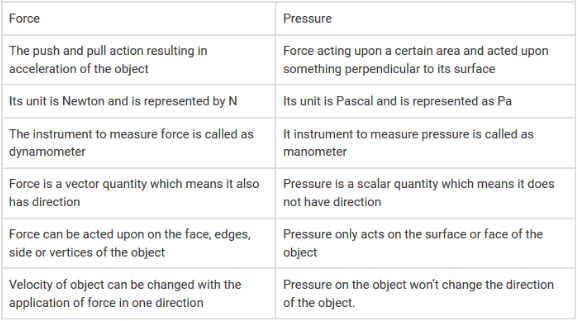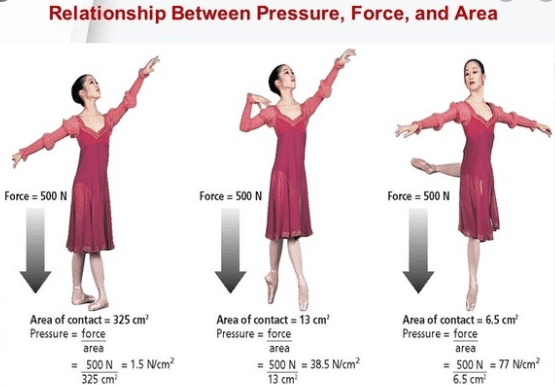Mechanics

# Difference between force and pressureThe difference between force and pressure is that “Force is an agency which moves or tends to move, stops or tends to stop the motion of a body. Force is the vector quantity. While pressure is the force acting normally per unit area on the surface of a body. It is a scalar quantity.

## Relation between pressure and forceMathematically, pressure can be written and calculated as:
Pressure = Force/Area
P =F/A
It means greater will be the force greater will be the pressure.

## Difference between force and pressure in tabular form

 Force Pressure Force is the push and pulls of an object. Pressure the force applying normally per unit area. Its SI unit is “Newton” denoted by N. Its SI unit is Pascal, denoted by “pa”. It is measured by spring balance or dynamo-meter. It is measured by Monometer. It is a vector quantity. It is a scalar quantity. It applies to faces, vertices, and edges. It applies to faces only. Its velocity can be changed. Its velocity cannot be changed.